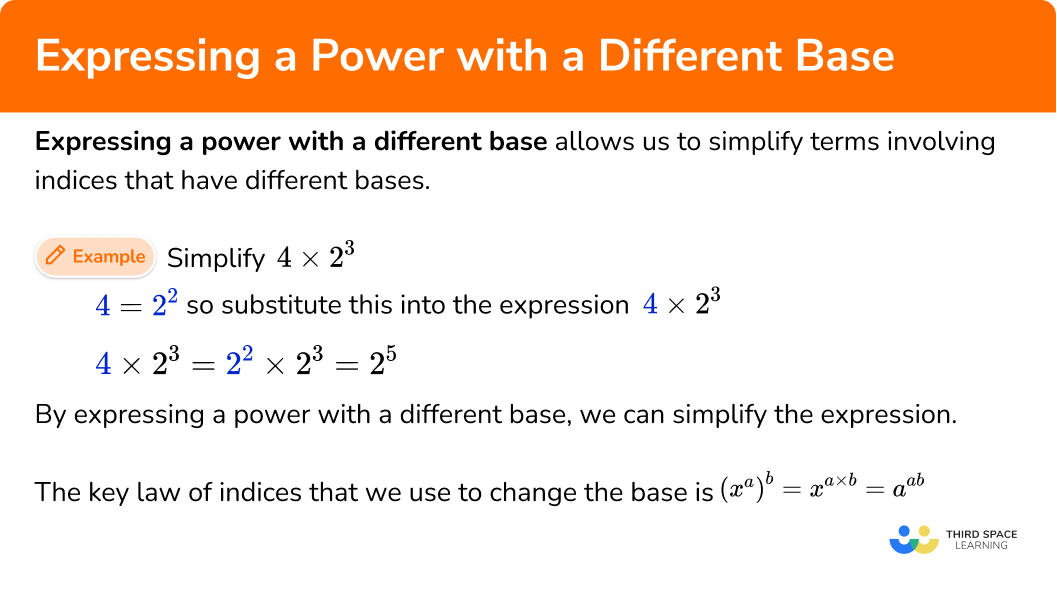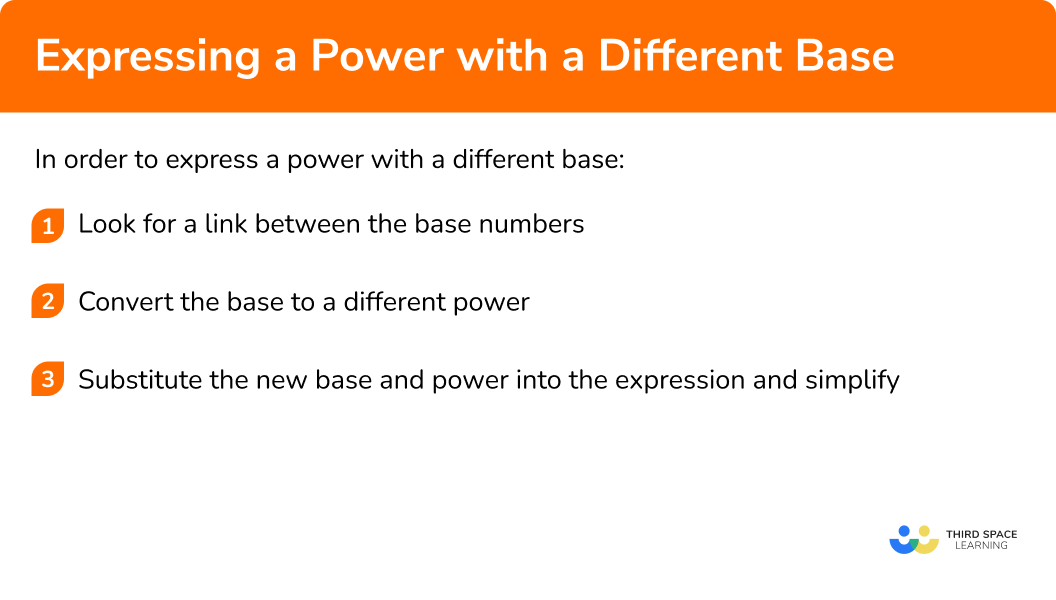GCSE Maths Number Powers And Roots

Expressing Powers

# Expressing A Power With A Different Base

Here we will learn about expressing a power with a different base.

There are also powers and roots worksheets based on Edexcel, AQA and OCR exam questions, along with further guidance on where to go next if you’re still stuck.

## What is expressing a power with a different base?

Expressing a power with a different base is simplifying terms involving indices that have different bases.

For example,

Simplify 4\times2^{3} .

As 4=2^{2} , we can substitute this into the expression 4\times2^{3} to get 2^{2}\times 2^{3} .

4\times 2^{3} = 2^{2}\times 2^{3}= 2^5

So by expressing a power with a different base, we can simplify the expression.

The key law of indices that we use to change the base is (x^{a})^{b}=x^{a\times b}=x^{ab} .

### What is expressing a power with a different base?## How to express a power with a different base

In order to express a power with a different base:

1. Look for a link between the base numbers.
2. Convert the base to a different power.
3. Substitute the new base and power into the expression and simplify.

### Explain how to express a power with a different base### Related lessons onpowers and roots

Expressing a power with a different base is part of our series of lessons to support revision on powers and roots. You may find it helpful to start with the main powers and roots lesson for a summary of what to expect, or use the step by step guides below for further detail on individual topics. Other lessons in this series include:

## Expressing a power with a different base examples

### Example 1: simplifying by changing the base

Simplify the expression 3^{4}\times 9^{2} .

1. Look for a link between the base numbers.

3 and 9 are both powers of 3 .

2Convert the base to a different power.

As 9=3^{2} , we can use this to change the base of 9 to a base of 3 .

3Substitute the new base and power into the expression and simplify.

As 9=3^{2} , we can now say that 3^{4}\times 9^{2} is the same as 3^{4}\times (3^{2})^{2} .

Simplifying this we get 3^{4}\times(3^{2})^{2}=3^{4}\times3^{2\times2}=3^{4}\times3^{4}=3^{4+4}=3^{8} .

So 3^{4}\times9^{2}=3^{8} .

### Example 2: algebraic

Simplify the following expression into the form a^{b}x^{b} where a and b are integers.

(4x^2)^{3}\div2x .

We can just look at the coefficients of x for this example.

4 is equal to 2^2 we can substitute this into the expression

4=2^{2}

As 4=2^{2} , we can substitute this into the expression to get,

(4x^2)^{3}\div2x=((2^2)x^2)^{3}\div2x=2^{6}x^{6}\div2x=2^{5}x^{5} .

So (4x^2)^{3}\div2x=2^{5}x^{5} .

### Example 3: multiple different bases

Evaluate the expression \frac{8^{3}\times{4^{-1}}}{16} .

4, 8 and 16 are all different powers of 2 and so we can convert them all to simplify the expression.

8=2^{3}

4=2^{2}

16=2^{4}

We can substitute these into the expression to get

\frac{8^{3}\times{4^{-1}}}{16}=\frac{(2^{3})^{3}\times{(2^{2})^{-1}}}{(2^{4})}

=\frac{2^{3\times{3}}\times{2^{2\times{-1}}}}{2^{4}}

=\frac{2^{9}\times{2^{-2}}}{2^{4}}

=2^{9+-2-4}=2^{9-2-4}=2^{3} .

As the question asks us to evaluate the expression, we get \frac{8^{3}\times{4^{-1}}}{16}=2^{3}=8 .

### Example 4: negative fractional power

Express the following as a single power of 5 .

125^{-\frac{2}{3}}

We only have one term however we have been told to express this as a power of 5 and so we can change 125 to be a power of 5 .

125=5^{3}

We can substitute these into the expression to get

125^{-\frac{2}{3}}=(5^{3})^{-\frac{2}{3}}=5^{3\times{-\frac{2}{3}}}=5^{-2} .

### Example 5: solving equations

Given that y=x^{2} and x^{3}y^{2}=128 , calculate the possible values of x .

We can convert y to be written in terms of x as y=x^{2} .

We do not need to fulfil this step as this conversion is already given in the question.

We can substitute these into the expression to get

x^3(x^2)^2=x^3\times(x^2)^2=x^3\times x^{2\times2}=x^3\times x^4=x^7 .

Now we have x^{7}=128 and so calculating the seventh root of 128 , we get \sqrt{128}=2 .

So x=2 .

### Example 6: simplify and evaluate

Evaluate \sqrt{3^{4}}+27^{\frac{2}{3}} .

We can convert 27 to be a power of 3 .

27=3^{3}

We can substitute these into the expression to get

\sqrt{3^{4}}+27^{\frac{2}{3}}=\sqrt{3^{4}}+(3^{3})^{\frac{2}{3}}=\sqrt{3^{4}}+3^{3\times{\frac{2}{3}}}=\sqrt{3^{4}}+3^{2} .

As, \sqrt{3^{4}}=3^{\frac{4}{2}}=3^{2} , we can simplify further to get

\sqrt{3^{4}}=27^{\frac{2}{3}}=3^{2}+3^{2}=9+9=18 .

### Common misconceptions

• Incorrect base

A possible error is to alter base number without altering the power.

For example,

When converting (4^{2})^{3} to a power of 2 , the base number 4 is changed to a 2 and is incorrectly substituted as (2^{2})^3 .

The correct answer is ((2^{2})^{2})^{3} .

Using brackets can help to avoid this mistake.

• Negative powers and negative number

A common error is to think that a negative power suggests that the entire number is a negative number. This is incorrect because a negative power means that we are finding a positive reciprocal of the base.

For example, 2^{-4}=-2^{4}=-16 is incorrect.

The correct answer is 2^{-4}=\frac{1}{2^{4}}=\frac{1}{16} .

• Changing the base incorrectly

For example, 5^{3}\times2^{-4}=(5\times{2})^{3-4}=10^{-1}=\frac{1}{10} . This is incorrect as the bases have been multiplied together. The base must be the same when simplifying calculations with powers. The correct answer would be 5^{3}\times{2^{-4}}=5^{3}\times{\frac{1}{16}}=\frac{125}{16} .

• BIDMAS

When raising a base to a power, the power is associated with a variable or an expression.

For example, let’s look at 3x^{-2} .

Using BIDMAS we can see that, x is being raised to the power of -2 , and then is multiplied by 3 to get the answer \frac{3}{x^{2}} .

However a common error is to calculate 3x raised to the power of -2 , giving the answer \frac{1}{9x^{2}} which is incorrect. If this was the case, the question would be written as (3x)^{-2} .

### Practice expressing a power with a different base questions

1. Express the following as a single power of 5 , 5^{3}\times 25^{2} .

5^{5}5^{6}5^{12}5^{7}5^{3}\times 25^{2}=5^{3}\times (5^{2})^{2}=5^{3}\times 5^{4}=5^{7}

2. Which expression is equivalent to 8y^{3}(2y)^{2} ?

(16y)^{5}(2y)^{6}(2y)^{5}32y^{6}8y^{3}=(2y)^{3}

(2y)^{3}\times (2y)^{2}=(2y)^{3+2}=(2y)^{5}

3. Express the following as a single power of 3 \frac{81^{2}\times{3^{-4}}}{27} .

3^{-1}33^{0}3^{-3}\begin{aligned} &\frac{81^{2} \times 3^{-4}}{27}=\frac{\left(3^{4}\right)^{2} \times 3^{-4}} {3^{3}}=\frac{3^{8} \times 3^{-4}}{3^{3}} \\\\ &=3^{8+-4-3}=3^{8-4-3}=3^{1}=3 \end{aligned}

4. Evaluate 81^{\frac{3}{4}} .

60.75279381^{\frac{3}{4}}=(3^{4})^{\frac{3}{4}}=3^{4\times{\frac{3}{4}}}=3^{3}=27

5. Given that b=a^{-4} and a^{6}b=16 , calculate the value of b .

\frac{1}{8}-642\frac{1}{256}a^{6}b=a^{6}\times a^{-4}=a^{2}=16 so a=4 .

b=a^{-4}=\frac{1}{a^{4}}=\frac{1}{4^{4}}=\frac{1}{256} .

6. Evaluate (\frac{9}{25})^{\frac{1}{2}}\times{5^{3}} .

97530150(\frac{9}{25})^{\frac{1}{2}}\times{5^{3}}=(\frac{3^{2}}{5^{2}})^{\frac{1}{2}}\times{5^{3}}=\frac{3}{5}\times{5^{3}}=3\times{5^{2}}

=3\times 25=75

### Expressing a power with a different base GCSE questions

1. Circle the expressions that are equivalent to 2^{4} .

4^{2} \hspace{3mm} \sqrt{64} \hspace{3mm} 8^{\frac{4}{3}} \hspace{3mm} 8 ^{-2} \hspace{3mm} \frac{2^{5}\times{2^{7}}}{2^{3}}

(2 marks)

4^{2} \hspace{3mm} 8^{\frac{4}{3}}

(2)

2. (a) Write the number x=3\times 100^{3} in standard form.

(b) Hence find the value of y=2x^{2} . Write your answer in standard form.

(4 marks)

(a)

x=3\times 10^{6}

(1)

(b)

x^{2}=(3\times 10^{6})^{2}=9\times 10^{12}

(1)

y=2x^{2}=9\times 10^{12}\times 2 = 18\times 10^{12}

(1)

y=1.8\times 10^{13}

(1)

3. (a) Express 18 as a product of prime factors in index form.

(b) Use your answer to part a) and laws of indices to write the expression below as a single power of 3 .

\frac{18\times{9^{-4}}}{2\times{3^{6}}}

(5 marks)

(a)

18=2\times 3^{2}

(1)

(b)

\frac{2\times{3^{2}}\times{9^{-4}}}{2\times{3^{6}}}

(1)

\frac{3^{2}\times{(3^2)^{-4}}}{3^{6}}

(1)

\frac{3^{2-8}}{3^{6}}

(1)

3^{-6-6}=3^{-12}

(1)

## Learning checklist

You have now learned how to:

• Consolidate your numerical and mathematical capability from key stage 3 and extend your understanding of the number system to include powers and roots
• Calculate with roots, and with integer {and fractional} indices
• Simplify expressions involving sums, products and powers, including the laws of indices

## Still stuck?

Prepare your KS4 students for maths GCSEs success with Third Space Learning. Weekly online one to one GCSE maths revision lessons delivered by expert maths tutors.

Find out more about our GCSE maths tuition programme.